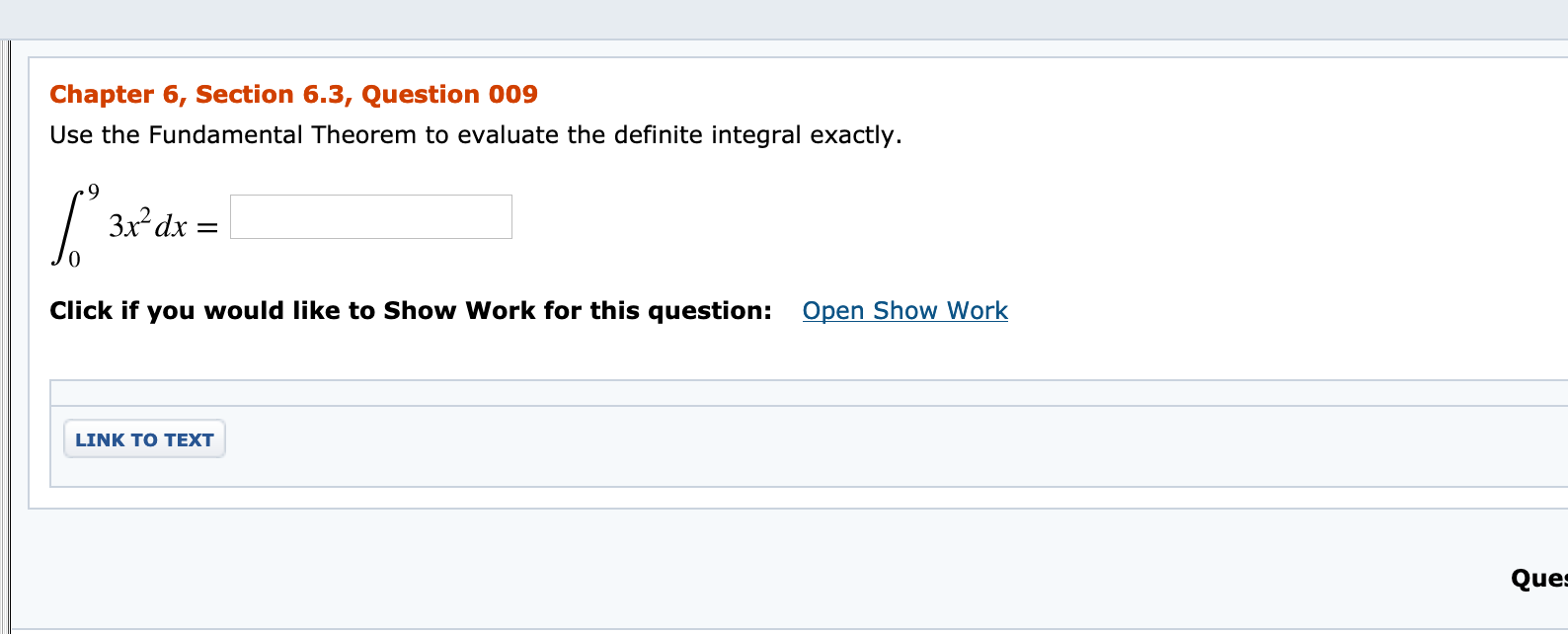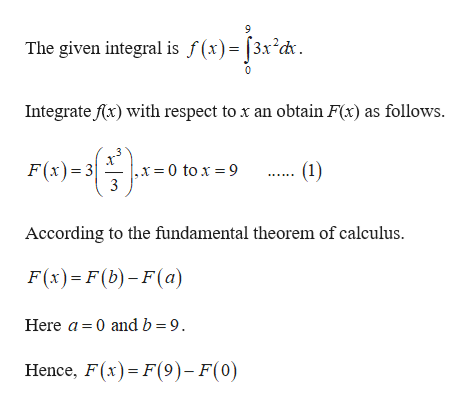# Chapter 6, Section 6.3, Question 009Use the Fundamental Theorem to evaluate the definite integral exactly.3x dx =Open Show WorkClick if you would like to Show Work for this question:LINK TO TEXTQues

Question
8 viewshelp_outlineImage TranscriptioncloseChapter 6, Section 6.3, Question 009 Use the Fundamental Theorem to evaluate the definite integral exactly. 3x dx = Open Show Work Click if you would like to Show Work for this question: LINK TO TEXT Ques fullscreen
check_circle

Step 1

Consider the given integ...help_outlineImage TranscriptioncloseThe given integral is f (x)= [3x²dx Integrate Ax) with respect to x an obtain F(x) as follows. F(x)= 3 .x=0 to x = 9 3 (1) According to the fundamental theorem of calculus. F(x)= F(b)– F(a) Here a = 0 and b = 9. Hence, F(x)=F(9)- F(0) fullscreen

### Want to see the full answer?

See Solution

#### Want to see this answer and more?

Solutions are written by subject experts who are available 24/7. Questions are typically answered within 1 hour.*

See Solution
*Response times may vary by subject and question.
Tagged in

### Integration